# Polyhedron facts for kids

Kids Encyclopedia Facts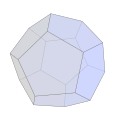Dodecahedron (Regular polyhedron)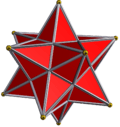Small stellated dodecahedron (Regular star)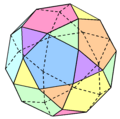Icosidodecahedron (Uniform)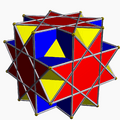Great cubicuboctahedron (Uniform star)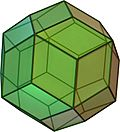Rhombic triacontahedron (Uniform dual)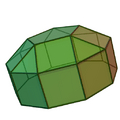Elongated pentagonal cupola (Convex regular-faced)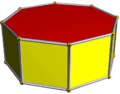Octagonal prism (Uniform prism)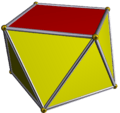Square antiprism (Uniform antiprism)

A polyhedron (one polyhedron, many polyhedra, or polyhedrons) is a geometrical shape. It has flat faces, and straight edges. Usually it is defined by the number of faces, or edges.

Mathematicians do not agree on what makes a polyhedron.

## Naming

Usually, polyhedra are named by the number of faces they have. The first polyhedra are the tetrahedron, which is made of four triangles, pentahedron (five faces, can look like a four-sided pyramid), hexahedron (six faces, a cube if it is regular), and heptahedron (seven faces, can look like a prism based on a pentagon, or a pyramid based on a hexagon amongst others).

## Images for kidsPolyhedron Facts for Kids. Kiddle Encyclopedia.